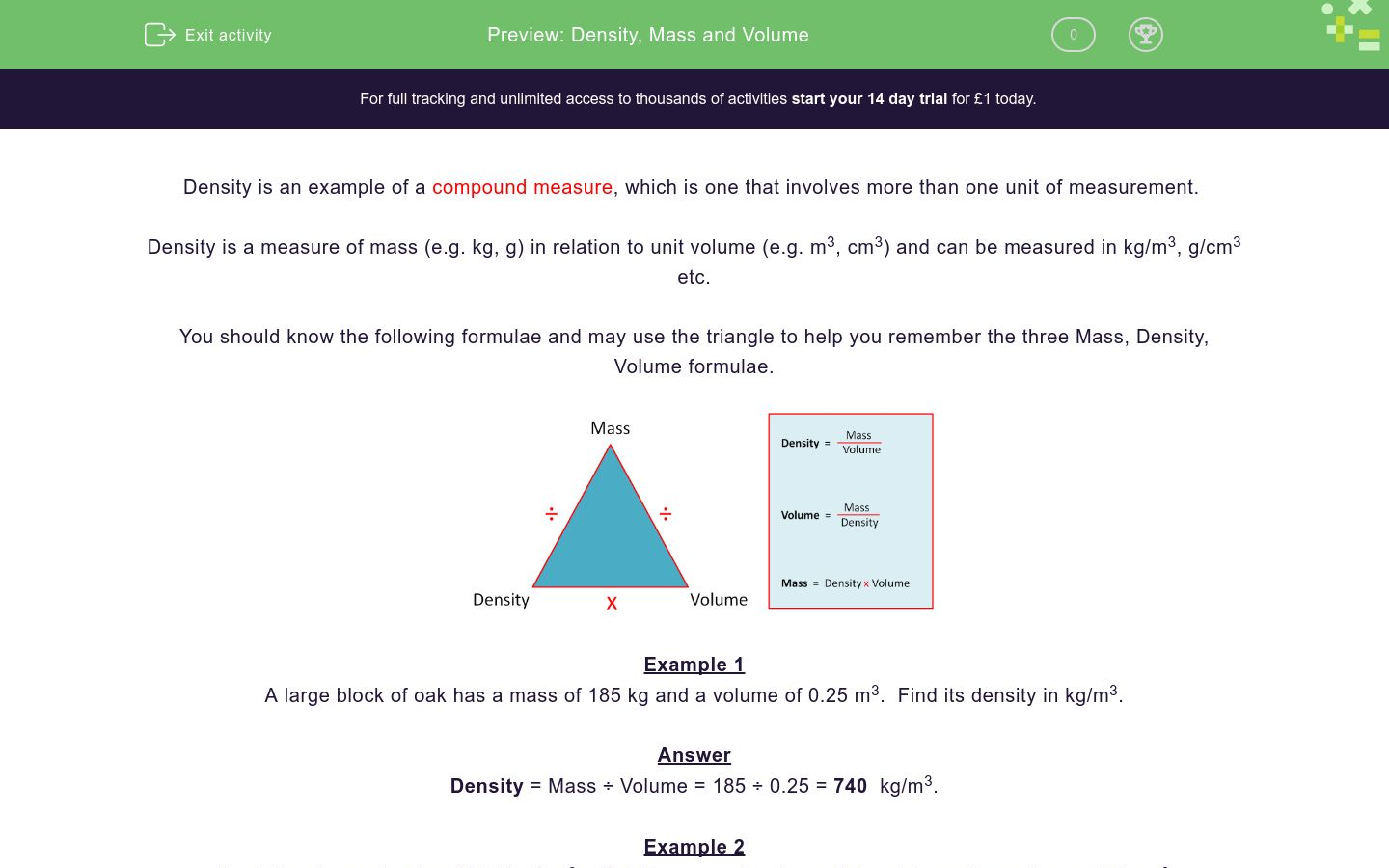# Density, Mass and Volume

In this worksheet, students work out densities, masses and volumes.Key stage:  KS 4

Curriculum topic:  Ratio, Proportion and Rates of Change

Curriculum subtopic:  Convert Between Related Compound Units

Difficulty level:### QUESTION 1 of 10

Density is an example of a compound measure, which is one that involves more than one unit of measurement.

Density is a measure of mass (e.g. kg, g) in relation to unit volume (e.g. m3, cm3) and can be measured in kg/m3, g/cm3 etc.

You should know the following formulae and may use the triangle to help you remember the three Mass, Density, Volume formulae.Example 1

A large block of oak has a mass of 185 kg and a volume of 0.25 m3.  Find its density in kg/m3.

Answer

Density = Mass ÷ Volume = 185 ÷ 0.25 = 740  kg/m3.

Example 2

Aluminium has a density of 2.712 g/cm3.  Find the mass of a piece of aluminium with a volume of 12 cm3.

Give your answer to 3 sig. figs.

Answer

Mass = Density x Volume

Mass = 2.712 x 12 = 32.5 g

A piece of chromium has a mass of 50g and a volume of 6.95 cm3.

Find the density of chromium in g/cm3.

(just write the number to 3 sig. figs.)

A piece of copper has a mass of ½ kg and a volume of 56 cm3.

Find the density of copper in g/cm3.

(just write the number to 3 sig. figs.)

The density of water is 1g/cm3.

Find the mass of 550 cm3 of water in grams.

(just write the number to 3 sig. figs.)

The density of iron is 7.85 g/cm3.

Find the volume in cm3 of 500g of iron.

(just write the number to 3 sig. figs.)

The density of gold is 19.32g/cm3.

Find the mass in grams of a piece of gold with volurme 50 cm3.

(just write the number to 3 sig. figs.)

The density of silver is 10.49 g/cm3.

Find the volume in cm3 of a 300g piece of silver.

(just write the number to 3 sig. figs.)

A piece of platinium has a mass of 192.6 g and a volume of 9 cm3.

Find the density of platinum in g/cm3.

(just write the number to 3 sig. figs.)

120m3 of fuel is poured into the tanks of an aeroplane.  The mass of the aeroplane increases by 88440 kg.

Find the density of the fuel in kg/m3.

(just write the number to 3 sig. figs.)

The density of milk is 1.02 g/cm3.

Find the mass in grams of a litre of milk.

(just write the number to 3 sig. figs.)

The density of a rock is 11.5 g/cm3 and it has a mass of ½ kg.

Find its volume in cm3.

(just write the number to 3 sig. figs.)

• Question 1

A piece of chromium has a mass of 50g and a volume of 6.95 cm3.

Find the density of chromium in g/cm3.

(just write the number to 3 sig. figs.)

CORRECT ANSWER
7.19
EDDIE SAYS
50 ÷ 6.95
• Question 2

A piece of copper has a mass of ½ kg and a volume of 56 cm3.

Find the density of copper in g/cm3.

(just write the number to 3 sig. figs.)

CORRECT ANSWER
8.93
EDDIE SAYS
500 ÷ 56
• Question 3

The density of water is 1g/cm3.

Find the mass of 550 cm3 of water in grams.

(just write the number to 3 sig. figs.)

CORRECT ANSWER
550
EDDIE SAYS
1 × 550
• Question 4

The density of iron is 7.85 g/cm3.

Find the volume in cm3 of 500g of iron.

(just write the number to 3 sig. figs.)

CORRECT ANSWER
63.7
EDDIE SAYS
500 ÷ 7.85
• Question 5

The density of gold is 19.32g/cm3.

Find the mass in grams of a piece of gold with volurme 50 cm3.

(just write the number to 3 sig. figs.)

CORRECT ANSWER
966
EDDIE SAYS
19.32 × 50
• Question 6

The density of silver is 10.49 g/cm3.

Find the volume in cm3 of a 300g piece of silver.

(just write the number to 3 sig. figs.)

CORRECT ANSWER
28.6
EDDIE SAYS
300 ÷ 10.49
• Question 7

A piece of platinium has a mass of 192.6 g and a volume of 9 cm3.

Find the density of platinum in g/cm3.

(just write the number to 3 sig. figs.)

CORRECT ANSWER
21.4
EDDIE SAYS
192.6 ÷ 9
• Question 8

120m3 of fuel is poured into the tanks of an aeroplane.  The mass of the aeroplane increases by 88440 kg.

Find the density of the fuel in kg/m3.

(just write the number to 3 sig. figs.)

CORRECT ANSWER
737
EDDIE SAYS
88440 ÷ 120
• Question 9

The density of milk is 1.02 g/cm3.

Find the mass in grams of a litre of milk.

(just write the number to 3 sig. figs.)

CORRECT ANSWER
1020
EDDIE SAYS
1.02 × 1000
• Question 10

The density of a rock is 11.5 g/cm3 and it has a mass of ½ kg.

Find its volume in cm3.

(just write the number to 3 sig. figs.)

CORRECT ANSWER
43.5
EDDIE SAYS
500 ÷ 11.5
---- OR ----

Sign up for a £1 trial so you can track and measure your child's progress on this activity.

### What is EdPlace?

We're your National Curriculum aligned online education content provider helping each child succeed in English, maths and science from year 1 to GCSE. With an EdPlace account you’ll be able to track and measure progress, helping each child achieve their best. We build confidence and attainment by personalising each child’s learning at a level that suits them.

Get started#### Similar activities you may like

Start your £1 trial today.
Subscribe from £10/month.